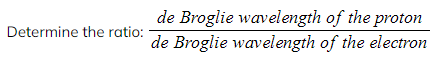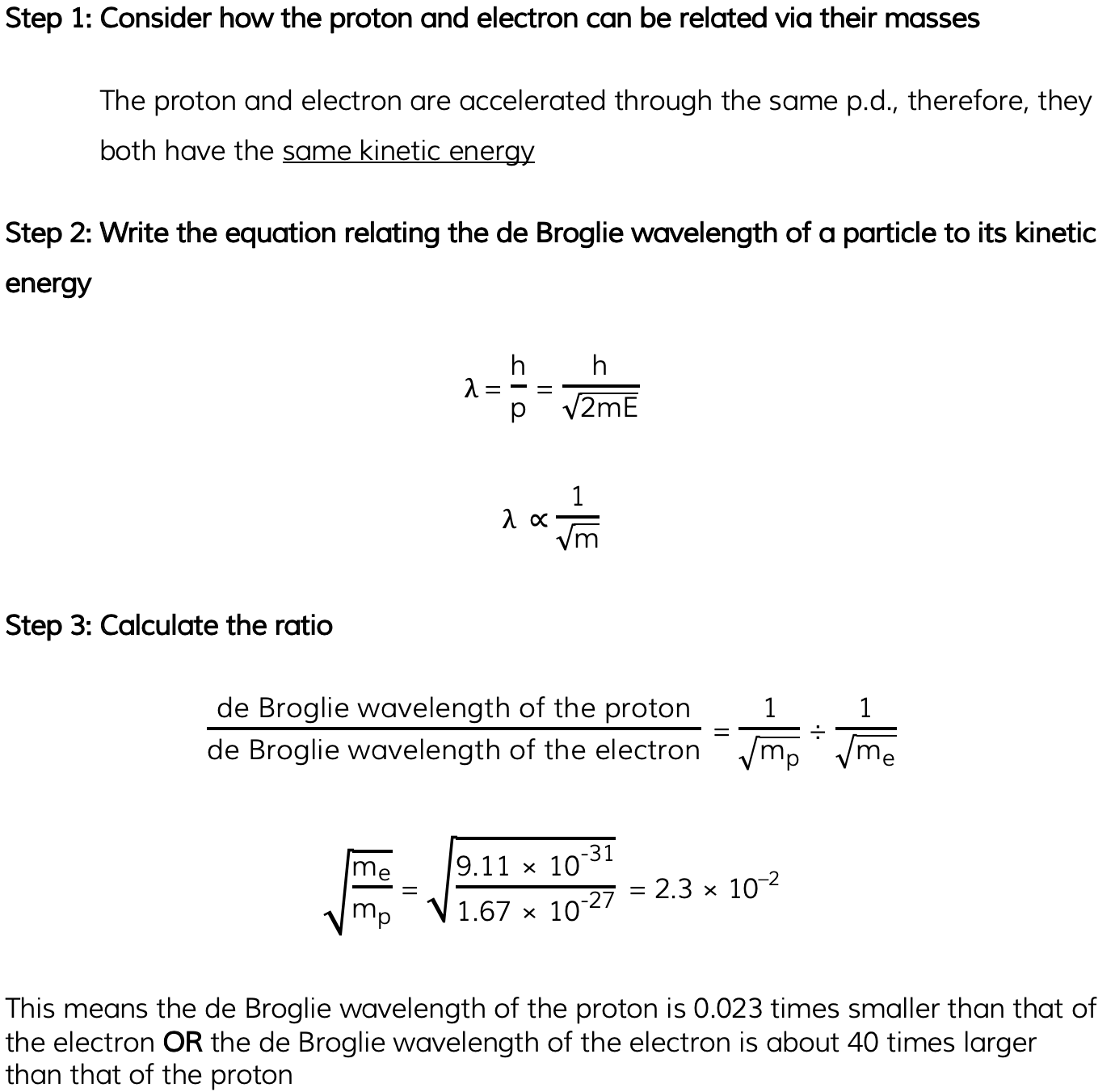# CIE A Level Physics复习笔记22.2.2 The de Broglie Wavelength

### The de Broglie Wavelength

• De Broglie proposed that electrons travel through space as a wave
• This would explain why they can exhibit behaviour such as diffraction
• He therefore suggested that electrons must also hold wave properties, such as wavelength
• This became known as the de Broglie wavelength
• However, he realised all particles can show wave-like properties, not just electrons
• So, the de Broglie wavelength can be defined as:

The wavelength associated with a moving particle

• The majority of the time, and for everyday objects travelling at normal speeds, the de Broglie wavelength is far too small for any quantum effects to be observed
• A typical electron in a metal has a de Broglie wavelength of about 10 nm
• Therefore, quantum mechanical effects will only be observable when the width of the sample is around that value
• The electron diffraction tube can be used to investigate how the wavelength of electrons depends on their speed
• The smaller the radius of the rings, the smaller the de Broglie wavelength of the electrons
• As the voltage is increased:
• The energy of the electrons increases
• The radius of the diffraction pattern decreases
• This shows as the speed of the electrons increases, the de Broglie wavelength of the electrons decreases

### Calculating de Broglie Wavelength

• Using ideas based upon the quantum theory and Einstein’s theory of relativity, de Broglie suggested that the momentum (p) of a particle and its associated wavelength (λ) are related by the equation:• Since momentum p = mv, the de Broglie wavelength can be related to the speed of a moving particle (v) by the equation:• Since kinetic energy E = ½ mv2
• Momentum and kinetic energy can be related by:• Combining this with the de Broglie equation gives a form which relates the de Broglie wavelength of a particle to its kinetic energy:• Where:
• λ = the de Broglie wavelength (m)
• h = Planck’s constant (J s)
• p = momentum of the particle (kg m s-1)
• E = kinetic energy of the particle (J)
• m = mass of the particle (kg)
• v = speed of the particle (m s-1)

#### Worked Example

A proton and an electron are each accelerated from rest through the same potential difference.• Mass of a proton = 1.67 × 10–27 kg
• Mass of an electron = 9.11 × 10–31 kg